# 使用TensorFlow编程实现一元逻辑回归

## 内容回顾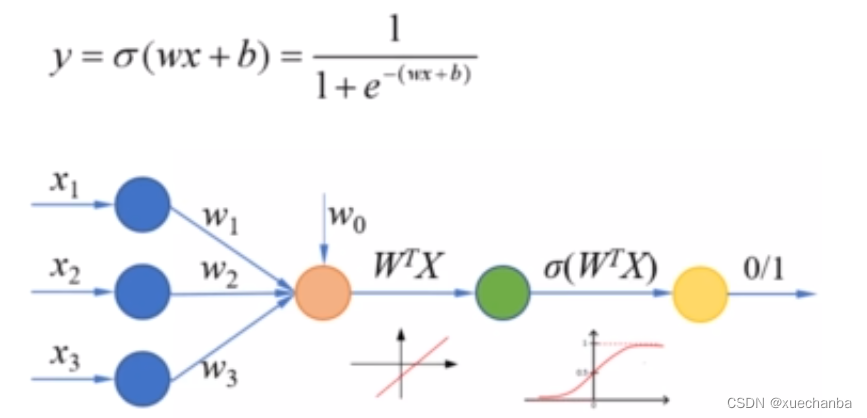## ; Sigmoid 函数：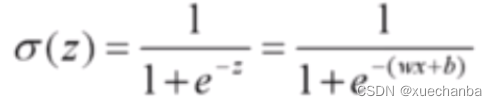``````import numpy as np
import tensorflow as tf

# 在TensorFlow中, 使用 exp 来实现 e^x 的运算,
# 需要注意这个函数的参数要求是浮点数。
x = np.array([1., 2., 3., 4.])

w = tf.Variable(1.)
b = tf.Variable(1.)

print(1/(1+tf.exp(-(w*x+b))))
"""
tf.Tensor([0.880797   0.95257413 0.98201376 0.9933072 ], shape=(4,), dtype=float32)
"""
``````

## 准确率：``````import numpy as np
import tensorflow as tf

# 假设 y 是样本标签
y = np.array([0, 0, 1, 1])
# pred 是对应的预测概率
pred = np.array([0.1, 0.2, 0.8, 0.49])

# 如果将阈值设置为 0.5 ,就可以使用四舍五入函数 round, 把它转换为 0 和 1
print(tf.round(pred))
"""
tf.Tensor([0. 0. 1. 0.], shape=(4,), dtype=float64)
"""

# 然后使用 equal 函数逐个元素的去比较预测值和标签值
print(tf.equal(tf.round(pred), y))
"""
tf.Tensor([ True  True  True False], shape=(4,), dtype=bool)
"""

# 使用 cast 函数将上面得到的结果转换为整数
print(tf.cast(tf.equal(tf.round(pred), y), tf.int8))
"""
tf.Tensor([1 1 1 0], shape=(4,), dtype=int8)
"""

# 得到预测正确的样本数在所有样本数中的比例
print(tf.reduce_mean(tf.cast(tf.equal(tf.round(pred), y), tf.float32)))
"""
tf.Tensor(0.75, shape=(), dtype=float32)
"""

# 需要注意的是, 使用 round 函数时, 如果参数恰好是0.5, 那么返回的结果是0.0
print(tf.round(0.5))
"""
tf.Tensor(0.0, shape=(), dtype=float32)
"""

# 当参数的值大于0.5时, 返回的结果才是是1.0
print(tf.round(0.5000001))
"""
tf.Tensor(1.0, shape=(), dtype=float32)
"""
``````

``````where (condition, a, b)
# 它根据condition返回a或者b的值，参数condition是一个布尔型的张量或者数组。
# 如果condition中的某个元素为真，那么对应位置就返回 a ，否则返回 b 。
``````

``````import numpy as np
import tensorflow as tf

# pred 是预测概率
pred = np.array([0.1, 0.2, 0.8, 0.49])

print(pred < 0.5)
# [ True  True False  True]

print(tf.where(pred < 0.5, 0, 1))
# tf.Tensor([0 0 1 0], shape=(4,), dtype=int32)

print(pred < 0.4)
# [ True  True False False]

print(tf.where(pred < 0.4, 0, 1))
# tf.Tensor([0 0 1 1], shape=(4,), dtype=int32)
``````

``````import numpy as np
import tensorflow as tf

# pred 是预测概率
pred = np.array([0.1, 0.2, 0.8, 0.59])

a = np.array([1, 2, 3, 4])
b = np.array([10, 20, 30, 40])

print(tf.where(pred < 0.5, a, b))
# tf.Tensor([ 1  2 30  40], shape=(4,), dtype=int32)

print(tf.where(pred >= 0.5))
# 当参数 a 和 b 省略时, 就返回数组 pred 中大小等于0.5的元素的索引
# 这个返回值以一个二维张量的形式给出
"""
tf.Tensor(
[
], shape=(2, 1), dtype=int64)
"""
# 这在我们需要获取某种条件的掩码时非常有用

# 在计算分类准确率时, 使用 where 函数可以更加灵活地设置阈值
# 然后将预测概率转化为类别
y = np.array([0, 0, 1, 1])
y_pred = np.array([0.1, 0.2, 0.8, 0.49])

print(tf.reduce_mean(tf.cast(tf.equal(tf.where(y_pred < 0.5, 0, 1), y), tf.float32)))
"""
tf.Tensor(0.75, shape=(), dtype=float32)
"""
``````

## 交差熵损失函数：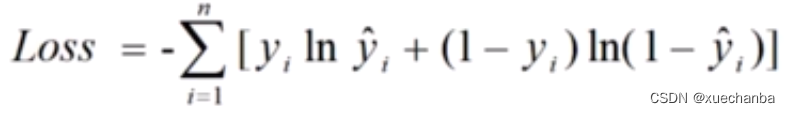## ; 平均交差熵损失函数：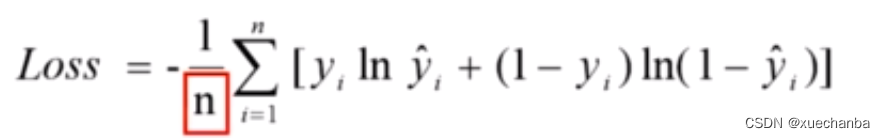``````import numpy as np
import tensorflow as tf

# 假设 y 是样本标签
y = np.array([0, 0, 1, 1])
# pred 是对应的预测概率
pred = np.array([0.1, 0.2, 0.8, 0.49])

# 计算交差熵损失函数值
# tf.math.log实现以 e 为底的对数运算
print(-tf.reduce_sum(y*tf.math.log(pred)+(1-y)*tf.math.log(1-pred)))
# tf.Tensor(1.2649975061637104, shape=(), dtype=float64)

# 计算平均交差熵损失函数值
print(-tf.reduce_mean(y*tf.math.log(pred)+(1-y)*tf.math.log(1-pred)))
# tf.Tensor(0.3162493765409276, shape=(), dtype=float64)
``````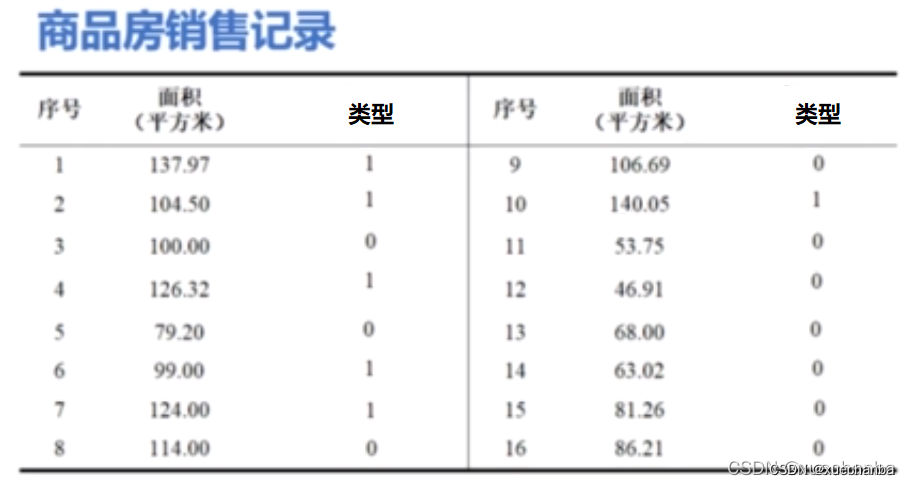（这个图中的数据比前面的不一样，修改了两个数据，目的是为了和其他相邻的点重合。其中，0代表普通住宅，1代表高档住宅。）

## 第一步：加载数据

``````import numpy as np
import tensorflow as tf

import matplotlib.pyplot as plt

plt.rcParams["font.family"] = "SimHei", "sans-serif"
plt.rcParams['axes.unicode_minus'] = False  # 设置正常显示符号

# 第一步：数据加载

# 面积都是大于40的正数
x = np.array([137.97, 104.50, 100.00, 126.32, 79.20, 99.00, 124.00, 114.00,
106.69, 140.05, 53.75, 46.91, 68.00, 63.02, 81.26, 86.21])

y = np.array([1, 1, 0, 1, 0, 1, 1, 0, 0, 1, 0, 0, 0, 0, 0, 0])

plt.figure()
plt.scatter(x, y)
plt.show()
``````（注：面积都是大于40的正数）

## 第二步：数据处理

``````x_train = x - np.mean(x)
y_train = y

plt.figure()
plt.scatter(x_train, y_train)
plt.suptitle("数据处理后的商品房分类图", fontsize=20)
plt.show()
``````## 第三步：设置超参数

``````learn_rate = 0.005
itar = 5
display_step = 1
``````

## 第四步：训练模型

``````cross_train = []  # 用来存放训练集的交叉熵损失
acc_train = []  # 用来存放训练集的分类准确率

for i in range(0, itar+1):

# Sigmoid 函数
pred_train = 1/(1+tf.exp(-(w*x_train+b)))
# 平均交叉熵损失函数
Loss_train = -tf.reduce_mean(y_train * tf.math.log(pred_train) + (1 - y_train) * tf.math.log(1 - pred_train))
# 计算准确率函数 -- 因为不需要对其进行求导运算, 因此也可以把这条语句写在 with 语句的外面
Accuarcy_train = tf.reduce_mean(tf.cast(tf.equal(tf.where(pred_train < 0.5, 0, 1), y_train), tf.float32))

# 记录每一次迭代的交叉熵损失和准确率
cross_train.append(Loss_train)
acc_train.append(Accuarcy_train)

# 对交叉熵损失函数分别对 w 和 b 求偏导
dL_dw, dL_db = tape.gradient(Loss_train, [w, b])

# 更新模型参数
w.assign_sub(learn_rate * dL_dw)
b.assign_sub(learn_rate * dL_db)

if i % display_step == 0:
print("i: %i, Train Loss: %f, Accuracy: %f" % (i, Loss_train, Accuarcy_train))
``````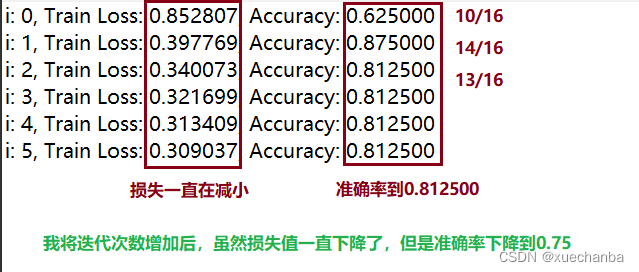## 第六步：数据可视化

1、绘制 Sigmoid 函数 曲线图

``````# 在设置模型初始值后, 给出一组连续的x坐标
x_ = range(-80, 80)
# 并使用 w 和 b 的初始值，计算Sigmoid 函数作为 y 坐标
y_ = 1/(1+tf.exp(-(w*x_+b)))
````````````# 在设置模型初始值后, 给出一组连续的x坐标
x_ = range(-600, 600)
# 并使用 w 和 b 的初始值，计算Sigmoid 函数作为 y 坐标
y_ = 1/(1+tf.exp(-(w*x_+b)))
``````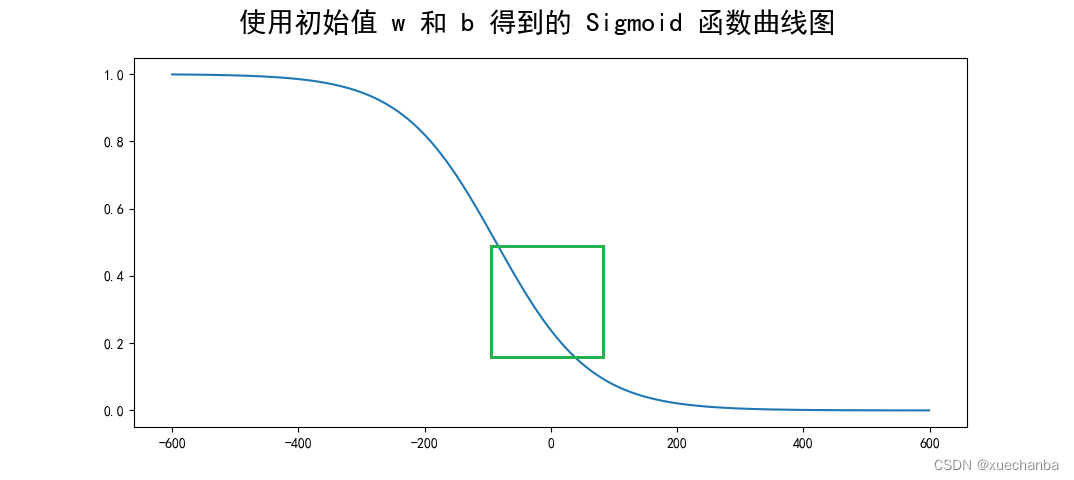``````# 第四步：设置模型变量的初始值
......

# 在设置模型初始值后, 给出一组连续的x坐标
x_ = range(-80, 80)
# 并使用 w 和 b 的初始值，计算Sigmoid 函数作为 y 坐标
y_ = 1/(1+tf.exp(-(w*x_+b)))

plt.figure()
# 绘制房子实际分类的散点图
plt.scatter(x_train, y_train)
plt.plot(x_, y_, color="red", linewidth=3)

# 第五步：训练模型
......

for i in range(0, itar+1):

......

......

if i % display_step == 0:
print("i: %i, Train Loss: %f, Accuracy: %f" % (i, Loss_train, Accuarcy_train))
y_ = 1/(1+tf.exp(-(w*x_+b)))
plt.plot(x_, y_)

plt.suptitle("不断更新 w 和 b 后得到的 Sigmoid 函数曲线图", fontsize=20)
plt.show()
``````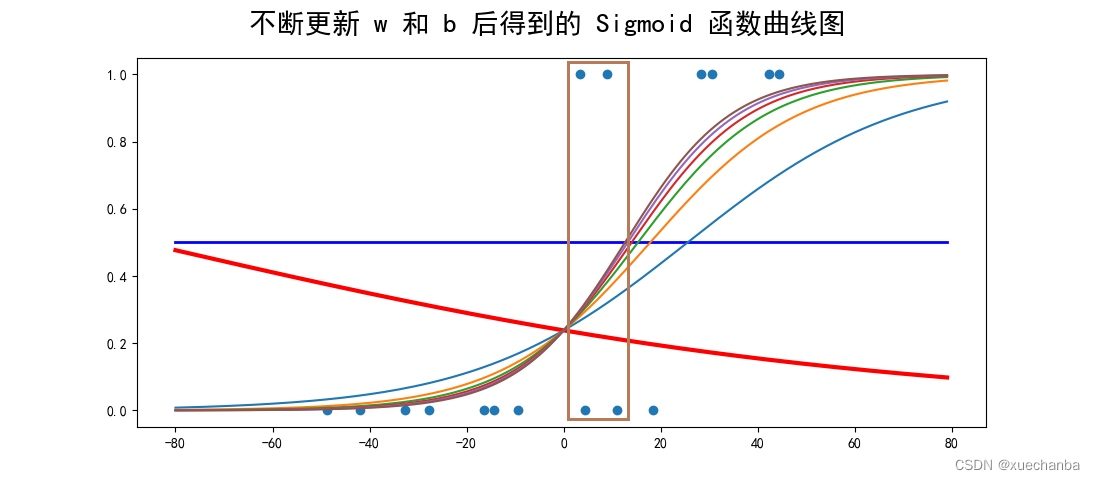## 第七步：模型预测

``````# 第七步：模型预测

x_test = np.array([128.15, 45.00, 141.43, 106.27, 99.00, 53.84, 85.36, 70.00, 162.00, 114.60])

# 这里使用训练数据的平均值对新的数据进行中心化处理
pred_test = 1 / (1 + tf.exp(-(w * (x_test-np.mean(x)) + b)))

# 根据概率进行分类
y_test = tf.where(pred_test < 0.5, 0, 1)

for i in range(len(x_test)):
print(x_test[i], "\t", pred_test[i].numpy(), "\t", y_test[i].numpy())
``````

``````"""
128.15   0.8610252       1
45.0     0.0029561974    0
141.43   0.9545566       1
106.27   0.45318928      0
99.0     0.2981362       0
53.84    0.00663888      0
85.36    0.108105935     0
70.0     0.028681064     0
162.0    0.9928677       1
114.6    0.6406205       1
"""
``````

``````plt.subplot(122)
plt.title("Test Outcome", fontsize=12)
plt.scatter(x_test, y_test)
x_ = np.array(range(-80, 80))
y_ = 1/(1 + tf.exp(-(w * x_ + b)))
plt.plot(x_+np.mean(x), y_)
plt.show()
``````Original: https://blog.csdn.net/xuechanba/article/details/124530357
Author: xuechanba
Title: 使用TensorFlow编程实现一元逻辑回归

## Title: dbpedia知识图谱java_一种基于DBpedia的水务领域概念知识图谱构建方法与流程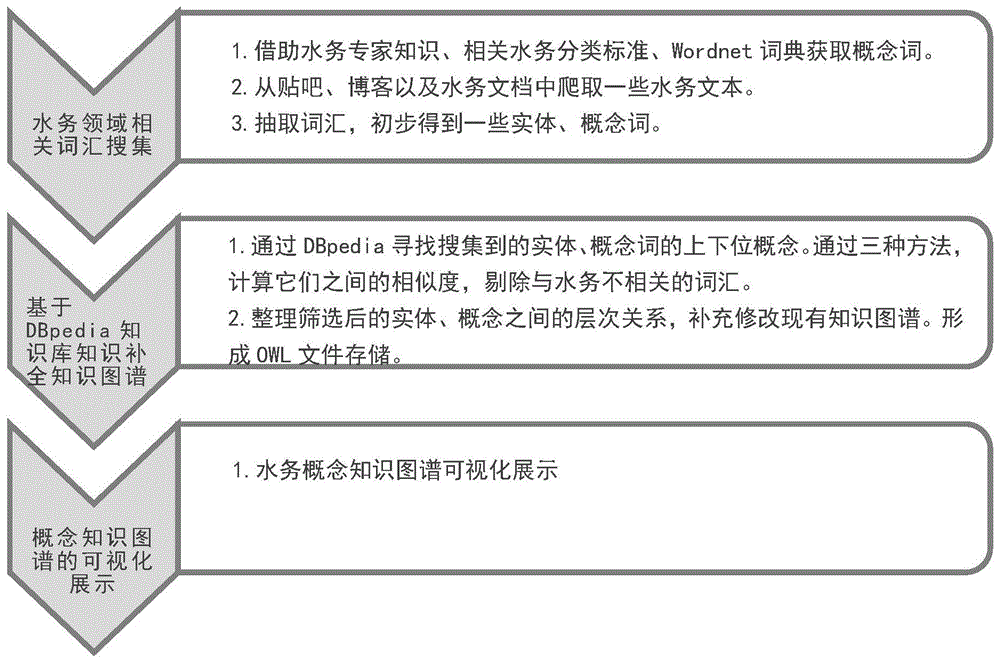1.本发明在水务实体、概念词抽取阶段，从大量的非结构文本中，能够抽取大量特定的水务实体、概念词，为水务知识图谱的构建打下坚实的基础。

2.本发明通过一定的技术方法，从DBpedia知识库中归类出水务领域的实体与概念，并将初步抽取的词汇进行了精确的筛选及关系的合并，并将获取的实体、概念关系作为现有知识图谱的补充，提供了一种知识图谱补全的方法。

Original: https://blog.csdn.net/weixin_39677203/article/details/114493090
Author: weixin_39677203
Title: dbpedia知识图谱java_一种基于DBpedia的水务领域概念知识图谱构建方法与流程